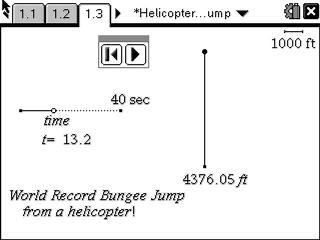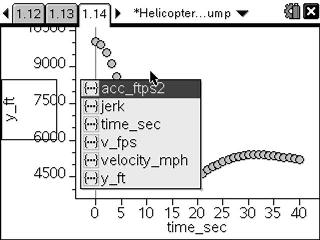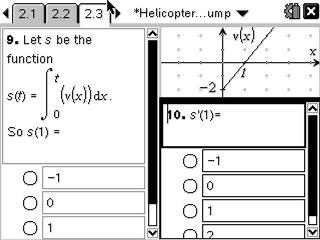# Activities

••• ##### Subject Area

• Math: Calculus: Applications of the Derivative
• Math: Calculus: Derivatives

• ##### Author9-12

40 Minutes

• ##### Device
• TI-Nspire™ Navigator™
• TI-Nspire™
• TI-Nspire™ CAS
• ##### Software

TI-Nspire™
TI-Nspire™ CAS

2.0

## Helicopter Bungee Jump#### Activity Overview

In this activity, students will observe a simulation of a record breaking bungee jump, consider a mathematical model of the height as a function of time, and take the derivative to determine points of interest like the minimum height, maximum velocity, acceleration, and maximum jerk. Students will investigate higher order derivatives.

#### Key Steps

•In this activity, students will observe a simulation of a record breaking bungee jump, consider a mathematical model of the height as a function of time, and take the derivative to determine points of interest like the minimum height, maximum velocity, acceleration, and maximum jerk. Students will algebraically, numerically, graphically and verbally investigate higher order derivatives

•Next, students will compare and contrast the position, velocity, acceleration, and jerk graphs.

•Self-check multiple choice questions enable students to get immediate feedback on their solution. Students also practice test questions relating a graph of a derivative to the original function. Students relate the characteristics of the graph of the first three derivatives of the original function.